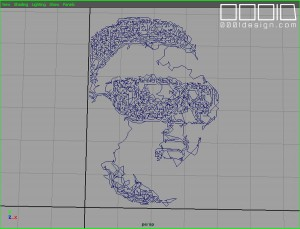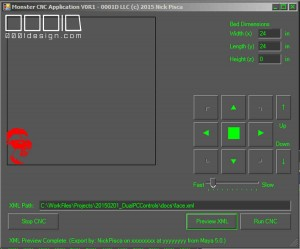This is a pretty simple MEL script we wrote called “xmllineGen.mel”.  It takes any series of one-degree straight lines in Maya (given they are lined up end to end) and extracts the endpoint XYZ vector, then saves them to an XML.  This conforms to the 0001D proprietary XML format that the CNC Monster application runs.  I should really post that spec…. Maybe once I test 3D options, then I can work out the bugs.

Task:  Going from Maya Lines to XML.

I have a 3D model with 2D lines in Maya like this:This script is so simple, it hardly requires a blog post, but here you go:

//0001D LLC 2015 (c) Nick Pisca

//XML Line Gen

string \$AllLines[] = `ls -tr “curve*”`;

\$exampleFileName = “C:\\WorkFiles\\Projects\\20150201_DualPCControls\\docs\\face.xml”;

int \$fileExists = `filetest -f \$exampleFileName`;

//if (\$fileExists == 0) {

//}

\$fileId=`fopen \$exampleFileName “w”`;   //print(\$fileId);

fprint \$fileId “<?xml version=\”1.0\” encoding=\”utf-8\”?>\r\n”;

fprint \$fileId (“<Export date=\”xxxxxxxx\” time=\”yyyyyyyy\” app=\”Maya 5.0\” author=\”NickPisca\” units=\”in\”>\r\n”);

fprint \$fileId (“<Path p=\”0\”>\r\n”);

for (\$x=0;\$x<size(\$AllLines);\$x++) {

print (“Exporting Lines… ” + \$x + ” of ” + size(\$AllLines) + “\n”);

vector \$PP = `pointPosition(\$AllLines[\$x]+”.cv”)`;

float \$xc = \$PP.x;

float \$yc = \$PP.y;

float \$zc = \$PP.z;

string \$xx = “\””+\$x+”\””;

string \$xs = “\””+\$xc+”\””;

string \$ys = “\””+\$yc+”\””;

string \$zs = “\””+\$zc+”\””;

string \$aa = “\”0.0\””;string \$LineStr = “<xyz n=” + \$xx + ” x=” + \$xs + ” y=” + \$ys + ” z=” + \$zs + ” o1=” + \$aa + ” o2=” + \$aa + ” o3=” + \$aa + “></xyz>”;

fprint \$fileId (\$LineStr+”\r\n”);

}

fprint \$fileId (“</Path>\r\n”);

fprint \$fileId (“</Export>\r\n”);

fclose \$fileId;

I didn’t have time to figure out how to get the date and time from Maya MEL (I know newer versions of AutoDesk Maya have this API, but I’m using 5.0 and 6.5).  Also, I could easily just write an exe or vbs or batch cmd line to extract the date and time, then load it with the command evaluation in MEL like this, but like I said, I’m just trying to test the efficacy of the CNC machine.  The only other noteworthy thing about this simple script is breaking down the string coordinates into separate variables.  For those of you who are new to programming and concatenation, especially when using those silly slash dual characters, it’s good to break this up.

Then upload the XML into the CNC Monster and voila!  The red lines on the left side confirm the image paths.Yay!  It works!